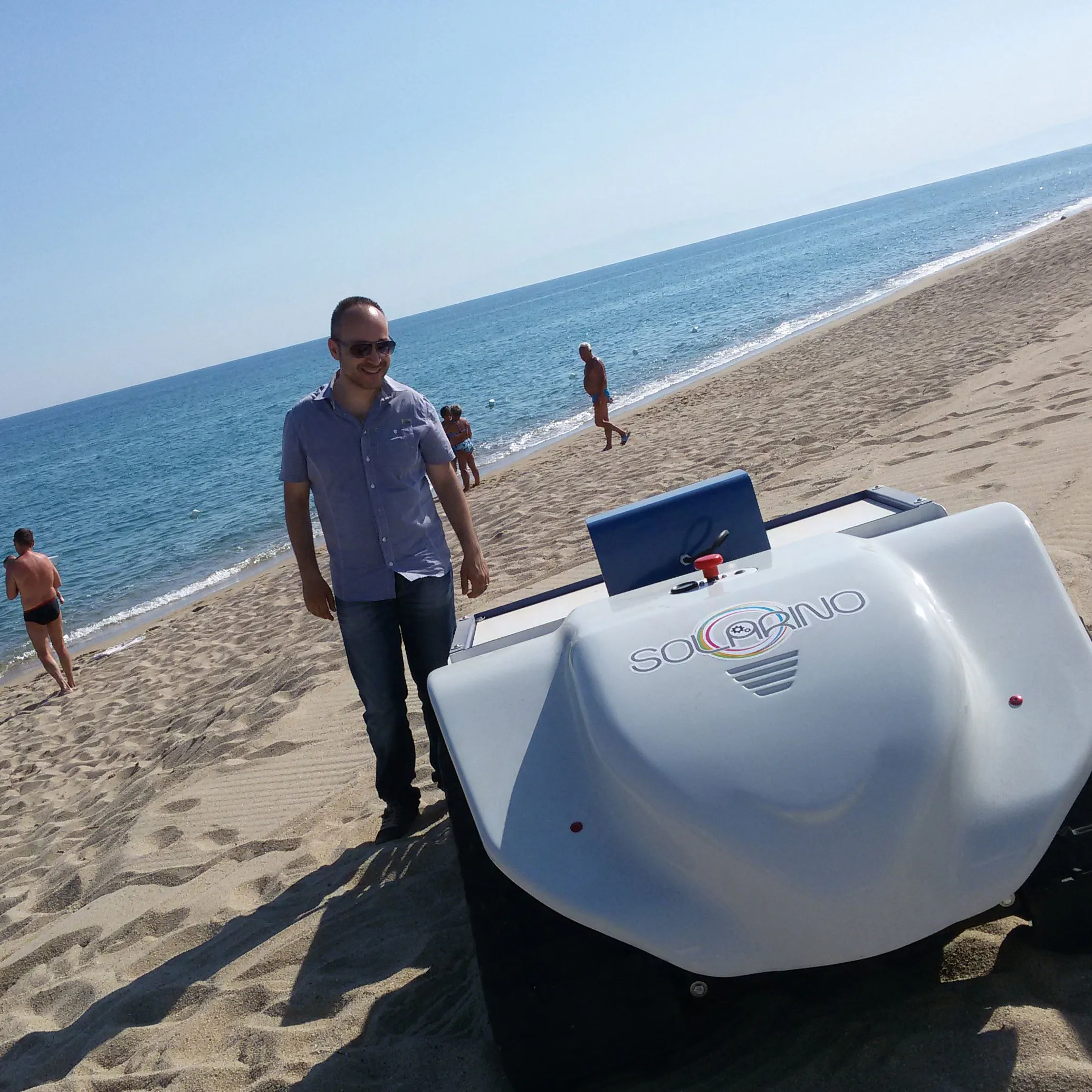# SoraBot-UVGI | Autonomous UVGI Robot

SoraBot-UVGI is a modular robot for sanitizing surfaces. It is small, it is equipped with self-driving, it is open-souce and low cost.

ExpertFull instructions provided5 days1,495

## Things used in this project

### Hardware components

 Cytron Technologies Cytron Power Window Motors w/ 5" Wheels (Pair)
×1
 Cytron Technologies MD20A - 20Amp 6V-30V DC Motor Driver
×2
 caster wheel - 40mm
×1
 GY-31 | TCS3200 Color Sensor Module
×2
 Line Follower - MH Sensor Module with TCRT5000L
×4Arduino Nano R3
×1Rechargeable Battery, 12 V Basic: Lead acid 12V 7Ah
×1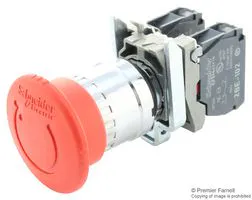Emergency Stop Switch, DPST It's a similar switch because I have used a recovery component.
×1
 LM2596 DC-DC Buck Converter Step-Down Power Module Output 1.25V-35V
×2
 Vishay Diode - BY500-600-E3
×1
 Geekcreit 310pcs 2.54mm Male Female Dupont Wire Jumper With Header Connector Housing Kit
×1
 Female Crimp Terminals
×1
 Philips hue Philips 62878740 36W 2G11 lampada UV
×2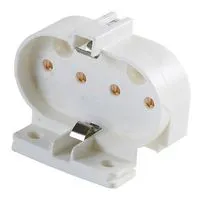Lamp Holder, 2G11 Compact Fluorescent Lamps
×2
 Electronic Ballast 220-240VAC 2x36W
×1
 Inverter 150W 12V 220V/230V
×1
 DSSERVO DS3225 25KG
×1
 TE Connectivity T92 Series Two-pole 30A Panel Mount Relay
×1
 Solid State Relays
×1
 Aluminum profile 20x20 I-type
×2
 selectable sliding block with web I-type - M4
×12
 selectable sliding block with web I-type - M5
×4

### Software apps and online servicesArduino IDEROS Robot Operating System

### Hand tools and fabrication machinesSoldering iron (generic)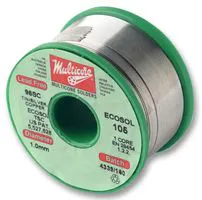PCB Holder, Soldering Iron3D Printer (generic)Drill / Driver, 20V
 Tool case

## Custom parts and enclosures

### SoraBot-UVGI base electronics

SoraBot-UVGI base electronics

### SoraBot-UVGI Emergency Button

SoraBot-UVGI Emergency Button

SoraBot-UVGI Top

### SoraBot-UVGI Cover Level 4

SoraBot-UVGI Cover Level 4

### SoraBot-UVGI | Support DS3225

SoraBot-UVGI | Support DS3225

### SoraBot-UVGI | Support DS3225 - 1

SoraBot-UVGI | Support DS3225 - 1

### SoraBot-UVGI | Support Lamp Holder

SoraBot-UVGI | Support Lamp Holder

### SoraBot-UVGI | Support Lamp

SoraBot-UVGI | Support Lamp

### SoraBot-UVGI | Support Lamp Reflector

SoraBot-UVGI | Support Lamp Reflector

## Schematics

### Drivers to Raspberry Pi 3

Electrical connection of two drivers to Raspberry Pi 3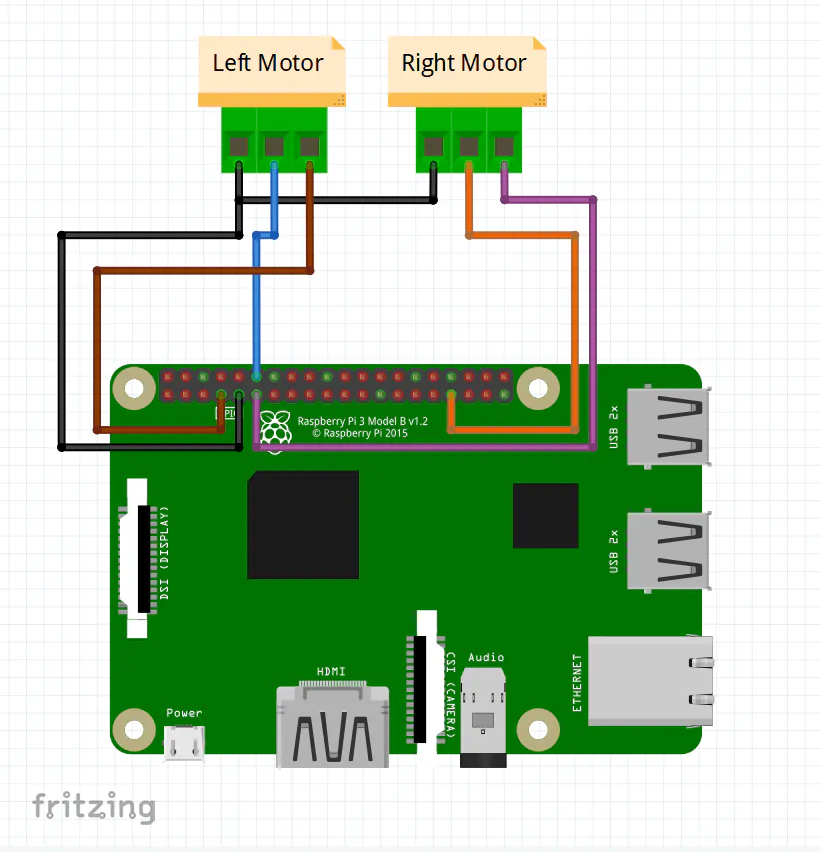### Power Supply

Electrical connection of power supply section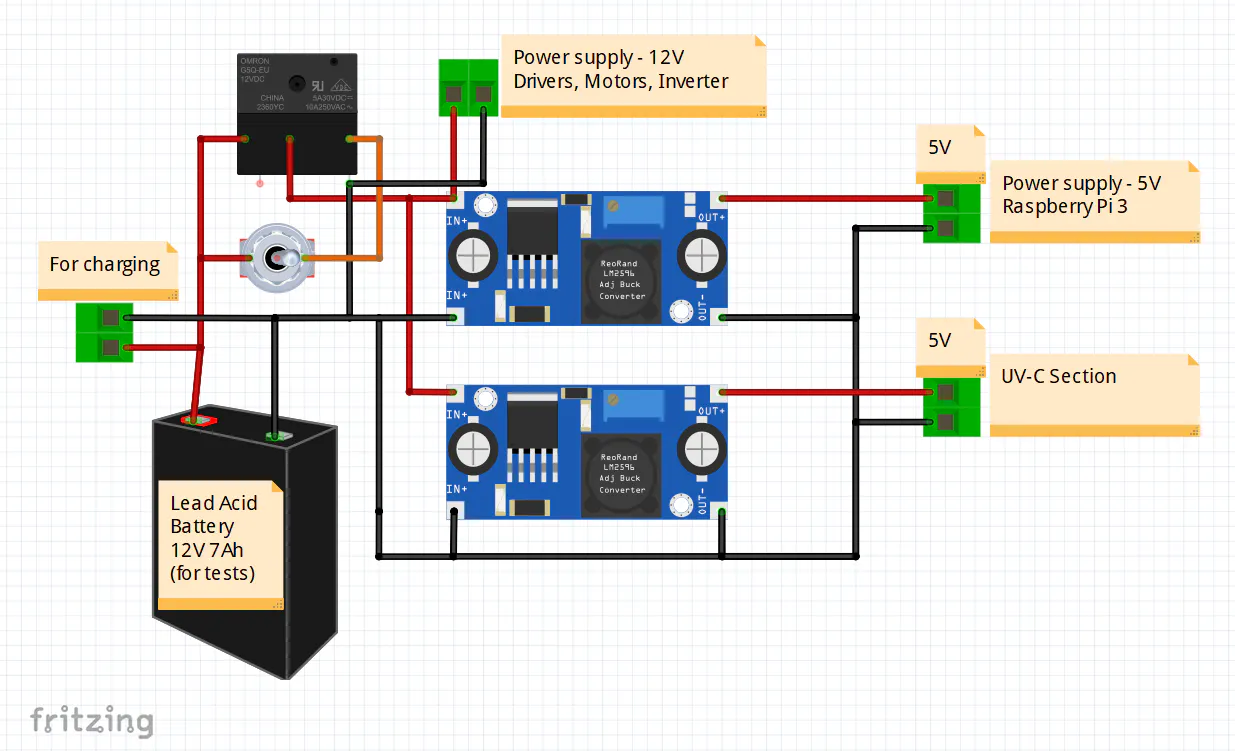### Line Followers

Electrical connection of line lollower sensors to Raspberry Pi 3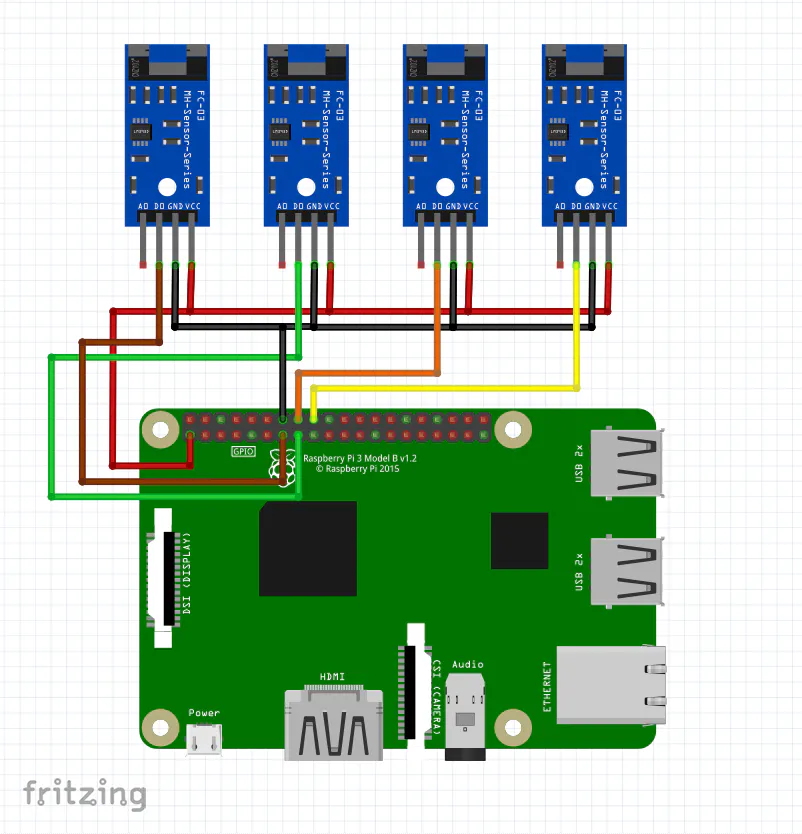### Color Sensors

Electrical connection of color sensors to Raspberry Pi 3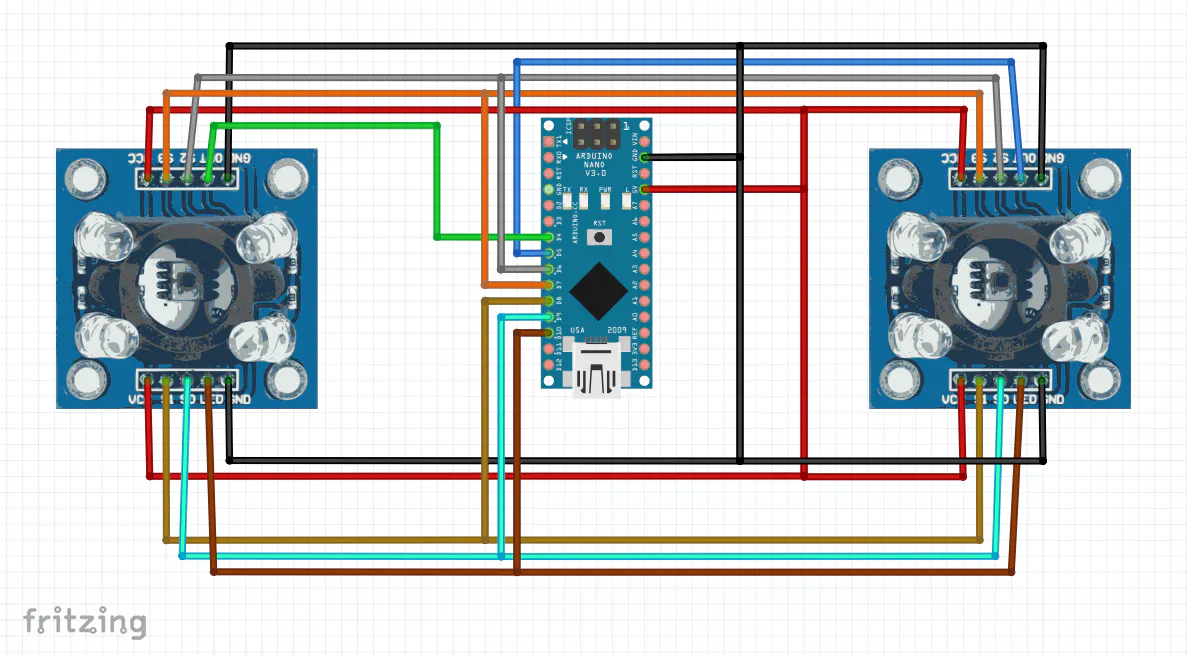### Ultrasonic Distance Sensors

Electrical connection of HC-SR04 ultrasonic distance sensors to Raspberry Pi 3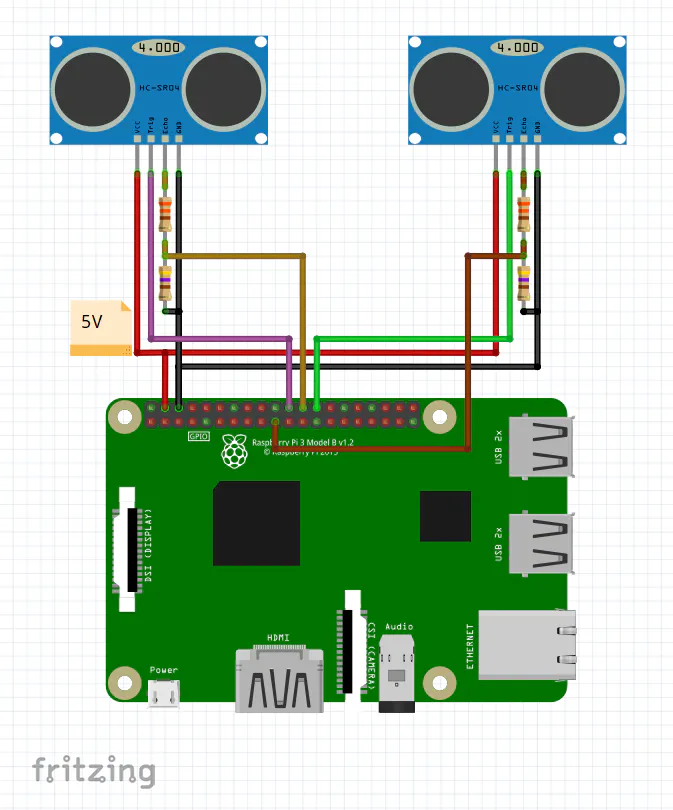### UV-C Section

Electrical schematic of UV-C section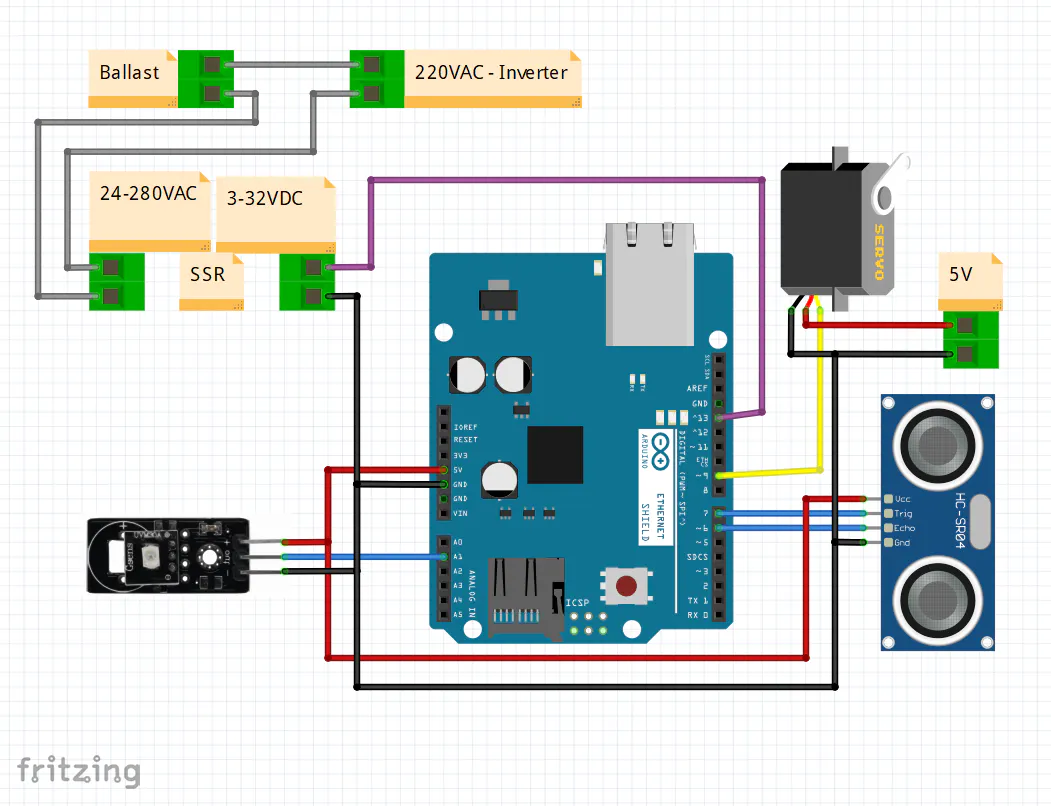## Code

### Code for reading color sensors with arduino nano

Arduino
The firmware is an Arduino sketch.
```// Mario Soranno
const int s0 = 9;         // Output frequency scaling selection
const int s1 = 8;         // Output frequency scaling selection
const int s2 = 6;         // Photodiode type selection
const int s3 = 7;         // Photodiode type selection
const int outSX = 4;      // Output frequency - SX: left Sensor Module
const int outDX = 5;      // Output frequency - SX: right Sensor Module
const int led = 10;       // white LEDs control
int brightness = 255;     // brightness of LEDs

int redSX = 0;            // Red value - SX: left Sensor Module
int greenSX = 0;          // Green value - SX: left Sensor Module
int blueSX = 0;           // Blue value - SX: left Sensor Module

int redDX = 0;            // Red value - DX: left Sensor Module
int greenDX = 0;          // Green value - DX: left Sensor Module
int blueDX = 0;           // Blue value - DX: left Sensor Module

void setup()
{
Serial.begin(9600);

pinMode(s0, OUTPUT);
pinMode(s1, OUTPUT);
pinMode(s2, OUTPUT);
pinMode(s3, OUTPUT);
pinMode(led, OUTPUT);
pinMode(outSX, INPUT);
pinMode(outDX, INPUT);

digitalWrite(s0, HIGH);
digitalWrite(s1, HIGH);
analogWrite(led, brightness);
}

void loop()
{
colors();
Serial.print("SX;");
Serial.print(redSX, DEC);
Serial.print(";");
Serial.print(greenSX, DEC);
Serial.print(";");
Serial.print(blueSX, DEC);

Serial.print(";DX;");
Serial.print(redDX, DEC);
Serial.print(";");
Serial.print(greenDX, DEC);
Serial.print(";");
Serial.println(blueDX, DEC);

delay(100);         // 100ms delay
}
void colors()
{
digitalWrite(s3, LOW);
redSX = pulseIn(outSX, digitalRead(outSX) == HIGH ? LOW : HIGH);    // Reads a pulse (either HIGH or LOW) on a pin.
redDX = pulseIn(outDX, digitalRead(outDX) == HIGH ? LOW : HIGH);    // Reads a pulse (either HIGH or LOW) on a pin.
digitalWrite(s3, HIGH);   // Blue value
blueSX = pulseIn(outSX, digitalRead(outSX) == HIGH ? LOW : HIGH);   // Reads a pulse (either HIGH or LOW) on a pin.
blueDX = pulseIn(outDX, digitalRead(outDX) == HIGH ? LOW : HIGH);   // Reads a pulse (either HIGH or LOW) on a pin.
digitalWrite(s2, HIGH);   // Green value
greenSX = pulseIn(outSX, digitalRead(outSX) == HIGH ? LOW : HIGH);  // Reads a pulse (either HIGH or LOW) on a pin.
greenDX = pulseIn(outDX, digitalRead(outDX) == HIGH ? LOW : HIGH);  // Reads a pulse (either HIGH or LOW) on a pin.
}
```

### colors - ROS Node

Python
ROS Node of color sensor - GY-31 Module
```#!/usr/bin/env python
import rospy
import serial
from sorabot.msg import colors_msg

# Mario Soranno

port = serial.Serial("/dev/ttyUSB0", baudrate=9600, timeout=3.0)

rv = ""
while True:
if ch != '\n':
rv += ch
if ch=='\r' or ch=='':
return rv

if __name__ == '__main__':
rospy.init_node('colors')
pub = rospy.Publisher("/colors_msg", colors_msg, queue_size = 10)
rate = rospy.Rate(10)
inc = 0
sxR = 0
sxG = 0
sxB = 0
dxR = 0
dxG = 0
dxB = 0
while not rospy.is_shutdown():
arrayric = rcv.split(";")
numelement = len(arrayric)
if numelement == 8:
arrayric = arrayric.replace('\r', '')
if arrayric == "SX" and arrayric == "DX":
sxR = int(arrayric)
sxG = int(arrayric)
sxB = int(arrayric)
dxR = int(arrayric)
dxG = int(arrayric)
dxB = int(arrayric)
inc = inc + 1

msg = colors_msg()
msg.inc = inc
msg.SX_Red = 0
msg.SX_Green = 0
msg.SX_Blue = 0
msg.DX_Red = 0
msg.DX_Green = 0
msg.DX_Blue = 0
# SX
if sxR < sxB and sxR < sxG and sxR < 20:
if sxR <=10 and sxG <=10 and sxB <=10:
msg.SX_Red = 0
msg.SX_Green = 0
msg.SX_Blue = 0
else:
msg.SX_Red = 1
msg.SX_Green = 0
msg.SX_Blue = 0
elif sxB < sxR and sxB < sxG:
if sxR <=10 and sxG <=10 and sxB <= 10:
msg.SX_Red = 0
msg.SX_Green = 0
msg.SX_Blue = 0
else:
msg.SX_Red = 0
msg.SX_Green = 0
msg.SX_Blue = 1
elif sxG < sxR and sxG < sxB:
if sxR <= 10 and sxG <=10 and sxB <= 10:
msg.SX_Red = 0
msg.SX_Green = 0
msg.SX_Blue = 0
else:
msg.SX_Red = 0
msg.SX_Green = 1
msg.SX_Blue = 0
else:
msg.SX_Red = 0
msg.SX_Green = 0
msg.SX_Blue = 0

# DX
if dxR < dxB and dxR < dxG and dxR < 20:
if dxR <=10 and dxG <=10 and dxB <=10:
msg.DX_Red = 0
msg.DX_Green = 0
msg.DX_Blue = 0
else:
msg.DX_Red = 1
msg.DX_Green = 0
msg.DX_Blue = 0
elif dxB < dxR and dxB < dxG:
if dxR <=10 and dxG <=10 and dxB <= 10:
msg.DX_Red = 0
msg.DX_Green = 0
msg.DX_Blue = 0
else:
msg.DX_Red = 0
msg.DX_Green = 0
msg.DX_Blue = 1
elif dxG < dxR and dxG < dxB:
if dxR <= 10 and dxG <=10 and dxB <= 10:
msg.DX_Red = 0
msg.DX_Green = 0
msg.DX_Blue = 0
else:
msg.DX_Red = 0
msg.DX_Green = 1
msg.DX_Blue = 0
else:
msg.DX_Red = 0
msg.DX_Green = 0
msg.DX_Blue = 0

pub.publish(msg)
rate.sleep()
```

### Drivers ROS Node

Python
ROS Node for control the drivers
```#!/usr/bin/env python
import rospy
import RPi.GPIO as GPIO
from sorabot.msg import drivers_msg

# Mario Soranno

if msg.direction == 0:
GPIO.output(7, GPIO.LOW)
GPIO.output(11, GPIO.LOW)
else:
GPIO.output(7, GPIO.HIGH)
GPIO.output(11, GPIO.HIGH)

if msg.rotation == 1:
pwm_left.start(msg.velocity)
pwm_right.start(msg.velocity+10)
elif msg.rotation == 3:
pwm_left.start(msg.velocity+10)
pwm_right.start(msg.velocity)
else:	# 2
pwm_left.start(msg.velocity)
pwm_right.start(msg.velocity)

if __name__ == '__main__':
rospy.init_node('drivers')
GPIO.setwarnings(False)
GPIO.setmode(GPIO.BOARD)	# This example uses the BOARD pin numbering
GPIO.setup(12, GPIO.OUT)
GPIO.setup(33, GPIO.OUT)
GPIO.setup(7, GPIO.OUT)
GPIO.setup(11, GPIO.OUT)
pwm_left = GPIO.PWM(12, 5000)
pwm_right = GPIO.PWM(33, 5000)
rospy.spin()
pwm_left.stop()
pwm_left.stop()
```

### Line Followers ROS Node

Python
ROS Node for read line follower sensors
```#!/usr/bin/env python
import rospy
from gpiozero import LineSensor
from sorabot.msg import linefollower_msg

# Mario Soranno

if __name__ == '__main__':
rospy.init_node('linefollower')
pub = rospy.Publisher("/linefollower", linefollower_msg, queue_size = 10)
rate = rospy.Rate(10)
inc = 0
followerSX = LineSensor(27)
followerSXC = LineSensor(22)
followerDXC = LineSensor(23)
followerDX = LineSensor(24)
while not rospy.is_shutdown():
inc = inc + 1
msg = linefollower_msg()
msg.inc = inc

if int(followerSX.value) == 0:
msg.followerSX = 0
else:
msg.followerSX = 1

if int(followerSXC.value) == 0:
msg.followerSXC = 0
else:
msg.followerSXC = 1

if int(followerDXC.value) == 0:
msg.followerDXC = 0
else:
msg.followerDXC = 1

if int(followerDX.value) == 0:
msg.followerDX = 0
else:
msg.followerDX = 1

pub.publish(msg)
rate.sleep()
```

### ultrasonic - ROS Node

C/C++
ROS Node for read ultrasonic sensors
```// Mario Soranno
#include "wiringPi.h"
#include "ros/ros.h"
#include "sorabot/ultrasonic_msg.h"

// Mario Soranno

int main(int argc, char **argv)
{
ros::init(argc, argv, "ultrasonic");
ros::NodeHandle node_obj;
sorabot::ultrasonic_msg msg;
ros::Rate loop_rate(10);
wiringPiSetup();
pinMode(6, OUTPUT);
pinMode(10, INPUT);
pinMode(11, OUTPUT);
pinMode(12, INPUT);
ros::Time begin = ros::Time::now();
ros::Time stop = ros::Time::now();
float distanceF = 0;
float distanceB = 0;
uint64_t difference = 0;
uint64_t TrigDuration = 0;
uint64_t inc = 0;
while(ros::ok())
{
distanceF = 0;
difference = 0;
TrigDuration = 0;
digitalWrite(6, HIGH);
begin = ros::Time::now();
while(TrigDuration < 12000)
{
stop = ros::Time::now();
TrigDuration = stop.toNSec() - begin.toNSec();
}
digitalWrite(6, LOW);
begin = ros::Time::now();
stop = ros::Time::now();
difference = stop.toNSec() - begin.toNSec();
distanceF = (difference * (340.0 / 1000000000.0)) / 2.0;

distanceB = 0;
difference = 0;
TrigDuration = 0;
digitalWrite(11, HIGH);
begin = ros::Time::now();
while(TrigDuration < 12000)
{
stop = ros::Time::now();
TrigDuration = stop.toNSec() - begin.toNSec();
}
digitalWrite(11, LOW);
begin = ros::Time::now();
stop = ros::Time::now();
difference = stop.toNSec() - begin.toNSec();
distanceB = (difference * (340.0 / 1000000000.0)) / 2.0;

inc++;
msg.inc = inc;
if(distanceF < 0.30) msg.ultrasonicF = 1;
else msg.ultrasonicF = 0;

if(distanceB < 0.30) msg.ultrasonicB = 1;
else msg.ultrasonicB = 0;

pub.publish(msg);
ros::spinOnce();
loop_rate.sleep();
}
return 0;
}
```

### kemet - ROS Node

Python
ROS Node for read kemet sensors
```#!/usr/bin/env python
import rospy
import serial
from sorabot.msg import kemet_msg

# Mario Soranno

port = serial.Serial("/dev/ttyACM0", baudrate=9600, timeout=3.0)

rv = ""
while True:
if ch != '\n':
rv += ch
if ch=='\r' or ch=='':
return rv

if __name__ == '__main__':
rospy.init_node('kemet')
pub = rospy.Publisher("/kemet", kemet_msg, queue_size = 10)
rate = rospy.Rate(10)
inc = 0
kemetF = 0
kemetB = 0
while not rospy.is_shutdown():
numelement = len(rcv)
msg = kemet_msg()
inc = inc + 1
msg.inc = inc
if numelement == 5:
msg.kemetF = int(rcv)
msg.kemetB = int(rcv)
else:
msg.kemetF = 0
msg.kemetB = 0

pub.publish(msg)
rate.sleep()
```

### Code for controlling UVC section with arduino Uno

Arduino
The firmware is an Arduino sketch.
```// Mario Soranno
#include <Servo.h>

// UVC Sensor
int sensorPin = A1;
int sensorValue = 0;

// HC-SR04
const int trigPin = 7;
const int echoPin = 6;
int distance; // variable for the distance measurement

// SERVO
Servo myservo;  // create servo object to control a servo
int pos = 0;    // variable to store the servo position

// SSR
const int ssr = 13;

void setup() {
Serial.begin(9600);
// SERVO
myservo.attach(9);  // attaches the servo on pin 9 to the servo object
myservo.write(10);
// HC-SR04
pinMode(trigPin, OUTPUT);
pinMode(echoPin, INPUT);
// SSR
pinMode(ssr, OUTPUT);
digitalWrite(ssr, LOW);
}

void loop() {
if (sensorValue > 40) Serial.println("UVC1");
else Serial.println("UVC0");

if (Serial.available()) {
switch (command)
{
case '0': // Vertical - UVC ON
for (pos = myservo.read(); pos >= 10; pos -= 1) {
myservo.write(pos);
delay(15);
}
digitalWrite(ssr, HIGH);
break;
case '1': // Horizontal - UVC ON
for (pos = myservo.read(); pos <= 100; pos += 1) {
myservo.write(pos);
distance = ultrasonic();
if(distance<20) distance = ultrasonic();
if(distance<20) break;
delay(15);
}
digitalWrite(ssr, HIGH);
break;
case '2': // Vertical - UVC OFF
for (pos = myservo.read(); pos >= 10; pos -= 1) {
myservo.write(pos);
delay(15);
}
digitalWrite(ssr, LOW);
break;
}
while (Serial.available()) {
}
}
delay(100);
}

int ultrasonic(void)
{
int dist;
long duration;
digitalWrite(trigPin, LOW);
delayMicroseconds(20);
digitalWrite(trigPin, HIGH);
delayMicroseconds(10);
digitalWrite(trigPin, LOW);
duration = pulseIn(echoPin, HIGH);
dist = (duration*0.034)/2;
return dist;
}
```

### uvcmodule - ROS Node

Python
ROS Node for control UV-C section
```#!/usr/bin/env python
import rospy
import serial
from std_msgs.msg import Int8
from sorabot.msg import uvcontrol_msg

# Mario Soranno

ser = serial.Serial("/dev/ttyUVC", baudrate=9600, timeout=3.0)

if msgrec.horizontal == 1 and msgrec.on == 1:
ser.write('1')
elif msgrec.horizontal == 0 and msgrec.on == 1:
ser.write('0')
else:
ser.write('2')

rv = ""
while True:
if ch != '\n':
rv += ch
if ch=='\r' or ch=='':
return rv

if __name__ == '__main__':
rospy.init_node('uvcmodule')
pub = rospy.Publisher("uvcvalue", Int8, queue_size = 10)
rate = rospy.Rate(10)
while not rospy.is_shutdown():
numelement = len(rcv)
msg = Int8()
if numelement == 5:
msg.data = int(rcv)
else:
msg.data = 9 	#error
pub.publish(msg)
rate.sleep()
```

### Save Data - ROS Node

Python
ROS node that save in a file the switching on and switching off times of the lamps.
```#!/usr/bin/env python
import rospy
import time
from sorabot.msg import uvcontrol_msg

# Mario Soranno

uvcon = 0
uvcon_last = 0

global uvcon
global uvcon_last

uvcon = msg.on
if uvcon != uvcon_last:
uvcon_last = uvcon
fileswitch = open("switchlamps.txt", "a")
if uvcon == 1:
now = time.strftime("%d/%m/%Y %H:%M:%S")
text = now + " - ON\n"
fileswitch.write(text)
else:
now = time.strftime("%d/%m/%Y %H:%M:%S")
text = now + " - OFF\n"
fileswitch.write(text)
fileswitch.close()

if __name__ == '__main__':
rospy.init_node('savedata')
rospy.spin()
```

### Guide - ROS Node

Python
ROS node that drives the robot.
```#!/usr/bin/env python
import rospy
from sorabot.msg import linefollower_msg
from sorabot.msg import drivers_msg
from sorabot.msg import ultrasonic_msg
from sorabot.msg import kemet_msg
from sorabot.msg import colors_msg
from std_msgs.msg import Int8
from sorabot.msg import uvcontrol_msg

# Mario Soranno

followerSX = 9
followerSXC = 9
followerDXC = 9
followerDX = 9

ultrasonicF = 9
ultrasonicB = 9

kemetF = 9
kemetB = 9

SX_Red = 9
SX_Green = 9
SX_Blue = 9
DX_Red = 9
DX_Green = 9
DX_Blue = 9

uvcvalue = 9

SXRcount = 0
SXGcount = 0
SXBcount = 0
DXRcount = 0
DXGcount = 0
DXBcount = 0

def callback_linefollower(msglinef):
global followerSX
global followerSXC
global followerDXC
global followerDX
followerSX = msglinef.followerSX
followerSXC = msglinef.followerSXC
followerDXC = msglinef.followerDXC
followerDX = msglinef.followerDX

def callback_ultrasonic(msgultra):
global ultrasonicF
global ultrasonicB

ultrasonicF = msgultra.ultrasonicF
ultrasonicB = msgultra.ultrasonicB

def callback_kemet(msgkemet):
global ultrasonicF
global ultrasonicB

kemetF = msgkemet.kemetF
kemetB = msgkemet.kemetB

def callback_colors(msgcolors):
global SX_Red
global SX_Green
global SX_Blue
global DX_Red
global DX_Green
global DX_Blue

global SXRcount
global SXGcount
global SXBcount
global DXRcount
global DXGcount
global DXBcount

if msgcolors.SX_Red == 1 and SXRcount < 2:
SXRcount = SXRcount + 1
elif msgcolors.SX_Red == 1 and SXRcount == 2:
SX_Red = 1
else:
SX_Red = 0
SXRcount = 0

if msgcolors.SX_Green == 1 and SXGcount < 2:
SXGcount = SXGcount + 1
elif msgcolors.SX_Green == 1 and SXGcount == 2:
SX_Green = 1
else:
SX_Green = 0
SXGcount = 0

if msgcolors.SX_Blue == 1 and SXBcount < 2:
SXBcount = SXBcount + 1
elif msgcolors.SX_Blue == 1 and SXBcount == 2:
SX_Blue = 1
else:
SX_Blue = 0
SXBcount = 0

if msgcolors.DX_Red == 1 and DXRcount < 2:
DXRcount = DXRcount + 1
elif msgcolors.DX_Red == 1 and DXRcount == 2:
DX_Red = 1
else:
DX_Red = 0
DXRcount = 0

if msgcolors.DX_Green == 1 and DXGcount < 2:
DXGcount = DXGcount + 1
elif msgcolors.DX_Green == 1 and DXGcount == 2:
DX_Green = 1
else:
DX_Green = 0
DXGcount = 0

if msgcolors.DX_Blue == 1 and DXBcount < 2:
DXBcount = DXBcount + 1
elif msgcolors.DX_Blue == 1 and DXBcount == 2:
DX_Blue = 1
else:
DX_Blue = 0
DXBcount = 0

def callback_uvcvalue(msguvc):
global uvcvalue

uvcvalue = msguvc.data

if __name__ == '__main__':
rospy.init_node('guide')
pub_drivers = rospy.Publisher("drivers", drivers_msg, queue_size = 10)
pub_uvcontrol = rospy.Publisher("uvcontrol", uvcontrol_msg, queue_size = 10)
sub_linefollower = rospy.Subscriber("linefollower", linefollower_msg, callback_linefollower)
sub_ultrasonic = rospy.Subscriber("ultrasonic", ultrasonic_msg, callback_ultrasonic)
sub_kemet = rospy.Subscriber("kemet", kemet_msg, callback_kemet)
sub_colors = rospy.Subscriber("colors", colors_msg, callback_colors)
sub_uvcvalue = rospy.Subscriber("uvcvalue", Int8, callback_uvcvalue)
msgdri = drivers_msg()
msguvc = uvcontrol_msg()
rate = rospy.Rate(10)
inc = 0
incuvc = 0
stop = 0
SX_Red_last = 0
SX_Green_last = 0
SX_Blue_last = 0
DX_Red_last = 0
DX_Green_last = 0
DX_Blue_last = 0
h_move = 0
while not rospy.is_shutdown():
incuvc = incuvc + 1
msguvc.inc = incuvc
inc = inc + 1
msgdri.inc = inc
msgdri.direction = 0

if uvcvalue_test == 1:	# -------- UV-C present --------
if ultrasonicF == 1 or ultrasonicB == 1 or kemetF == 1 or kemetB == 1:	# -------- pause --------
msgdri.rotation = 0
msgdri.velocity = 0
msguvc.on = 0 			# UV-C Lamps off - vertical
msguvc.horizontal = 0 	# vertical
else:	# Line follower
msgdri.velocity = 20
if followerDXC == 0:		# -------- turn left --------
msgdri.rotation = 1
if h_move == 0:
msguvc.on = 1 			# UV-C Lamps on - vertical
msguvc.horizontal = 0 	# UV-C Lamps on - vertical
stop = 0
elif followerSXC == 0:		# -------- turn right --------
msgdri.rotation = 3
if h_move == 0:
msguvc.on = 1 			# UV-C Lamps on - vertical
msguvc.horizontal = 0 	# UV-C Lamps on - vertical
stop = 0
elif followerSX == 1 and followerSXC == 1 and followerDXC == 1 and followerDX == 1:	# -------- stop --------
msgdri.rotation = 0
msgdri.velocity = 0
msguvc.on = 0 			# UV-C Lamps off - vertical
msguvc.horizontal = 0 	# vertical
stop = 1
else:
msgdri.rotation = 2	# go straight ahead
if h_move == 0:
msguvc.on = 1 			# UV-C Lamps on - vertical
msguvc.horizontal = 0 	# UV-C Lamps on - vertical
stop = 0

# Colors SX
if stop == 0 and SX_Red == 1 and SX_Red_last != 1:		# UVGI point - vertical
SX_Red_last = 1
msgdri.rotation = 0
msgdri.velocity = 0
pub_drivers.publish(msgdri)
msguvc.on = 1 						# UV-C Lamps off - vertical
msguvc.horizontal = 0 				# vertical
pub_uvcontrol.publish(msguvc)
rospy.sleep(12)						# 12 seconds
elif SX_Red == 0 and SX_Red_last == 1:
SX_Red_last = 0
elif stop == 0 and SX_Blue == 1:		# UVGI point - horizontal
SX_Blue_last = 1
msgdri.rotation = 0
msgdri.velocity = 0
pub_drivers.publish(msgdri)
msguvc.on = 1 						# UV-C Lamps on - vertical
msguvc.horizontal = 1 				# horizontal
pub_uvcontrol.publish(msguvc)
rospy.sleep(12)						# 12 seconds
elif SX_Blue == 0 and SX_Blue_last == 1:
SX_Blue_last = 0
elif stop == 0 and SX_Green == 1 and h_move == 0 and DX_Green_last == 0:	# UVGI point - horizontal in movement
DX_Green_last = 1
h_move = 1
msguvc.on = 1 			# UV-C Lamps on - vertical
msguvc.horizontal = 1 	# UV-C Lamps on - vertical
pub_uvcontrol.publish(msguvc)
elif SX_Green == 0 and DX_Green_last == 1:
DX_Green_last = 0
elif stop == 0 and SX_Green == 1 and h_move == 1 and DX_Green_last == 0:
DX_Green_last = 1
h_move = 0

else:	# -------- UV-C not present --------
msgdri.rotation = 0
msgdri.velocity = 0
msguvc.on = 0 				# UV-C Lamps off - vertical
msguvc.horizontal = 0 		# UV-C Lamps on - vertical

pub_uvcontrol.publish(msguvc)
pub_drivers.publish(msgdri)
rate.sleep()
```

## Credits

### Mario Soranno

9 projects • 21 followers
I have a Bachelor's Degree in Industrial Engineering - Electronics. I am an expert in hardware design, I can program in C/C++,Python and ROS